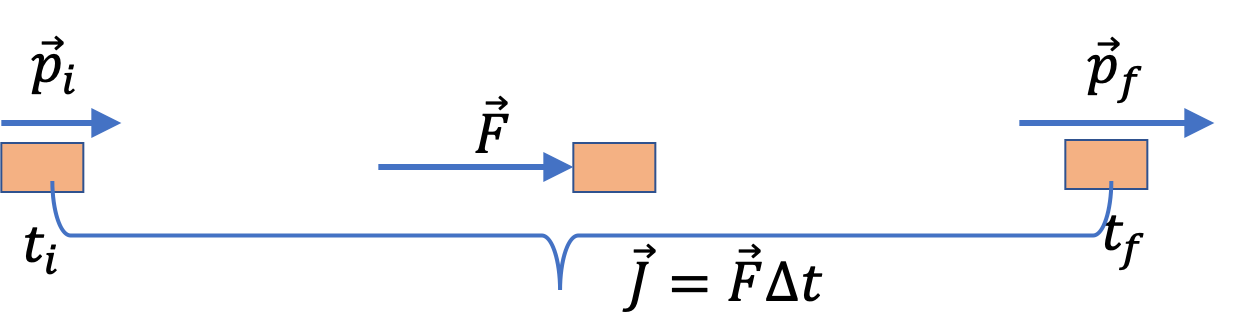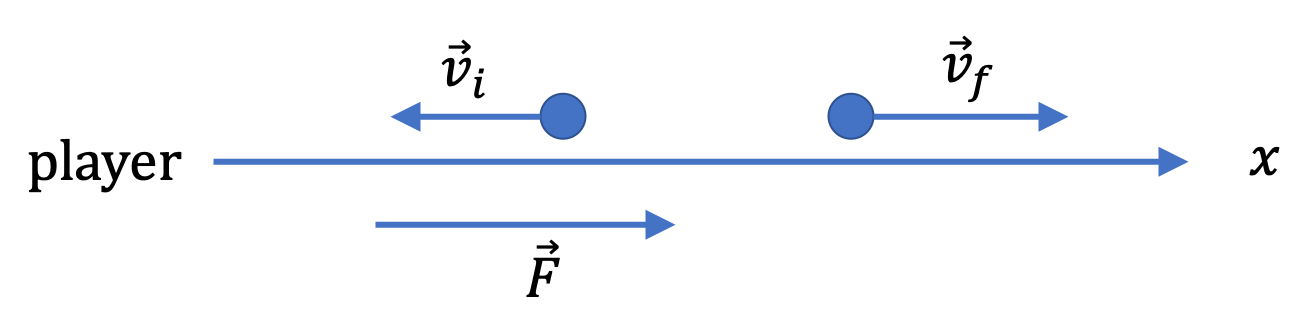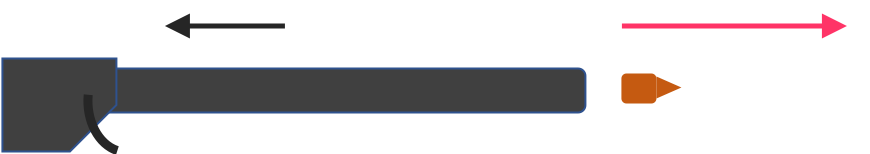## Section7.3Impulse and Change in Momentum

Recall that Newton's second law of motion, which was $\vec F = m\vec a$ for a system of unchanging mass, was special case of a more general expression in terms of rate of change of momentum.

\begin{equation} \vec F = \frac{\Delta \vec p}{\Delta t} \leftrightarrow \vec F = \frac{d \vec p}{d t}.\tag{7.3.1} \end{equation}

We can multiply both sides by $\Delta t$ to note that impulse $\vec J$ will equal change in momentum.

\begin{equation} \vec J = \vec F \Delta t = \Delta \vec p.\label{eq-change-in-momentum-equals-impulse}\tag{7.3.2} \end{equation}

This is illustrated in Figure 7.3.1.Figure 7.3.1. Each force causes a change in momentum equal to the impulse of the force, $\Delta \vec p = \vec J \text{,}$ where $\Delta \vec p = \vec p_f - \vec p_i$ and $\vec J$ is the impulse of the force.

Since Eq. (7.3.2) is a vector equation, the directions of the change in momentum and impulse vectors will be same, and their magnitudes will also be equal.

If multiple forces act on the body, impulse from each force will cause its own change in momentum. This is similar to the acceleration of the body caused by each force. The observed change in momentum will be the change in the total momentum, which is again similar to the observed acceleration as the total acceleration from all forces. For the case of $n$ forces on the body we would get the following changes caused by each impulse.

\begin{align*} \amp \Delta \vec p_1 = \vec J_1,\ \ \ \Delta \vec p_2 = \vec J_2,\ \ \ \cdots,\ \ \ \Delta \vec p_n = \vec J_n. \end{align*}

When we vectorially add all the impulses and all the changes in momenta, we find that the net impulse will give rise to the observed net change in momentum.

\begin{equation} \Delta \vec p_{\text{net}} = \vec J_{\text{net}},\label{eq-net-change-in-momentum-equal-net-impulse}\tag{7.3.3} \end{equation}

where

\begin{align*} \amp\Delta \vec p_{\text{net}} = \Delta\vec p_1 + \Delta\vec p_2 + \cdots + \Delta\vec p_n, \\ \amp \vec J_{\text{net}} = \vec J_1 + \vec J_2 + \cdots + \vec J_n. \end{align*}
###### Remark7.3.2.Impulse is defined by force and duration, not by momentum.

Eq. (7.3.2) is sometimes incorrectly interpreted as defining impulse. Impulse happens to equal change in momentum, just like, $\vec F = m \vec a$ does not mean force is $m\vec a\text{,}$ it just happes to equal $m\vec a\text{.}$ Force is a push or pull applied by some other body while $m\vec a$ is mass times acceleration of the body. Similarly, here, impulse is a concept related to force from other bodies and duration for which force acts, and not on momentum.

A player hits a $46\text{-gram}$ golf ball with an average force of $4,600\text{ N}$ while the golf club is in contact with the ball for $0.5\text{ ms}\text{.}$

Find the speed of the ball immediately after leaving the club if the golf ball was at rest before being it was struck.

Hint

Use $x$ axis only.

$50\text{ m/s}\text{.}$

Solution

Speed is the magnitude of velocity, which can be obtained by dividing the magnitude of momentum by mass. In general, to find the final momentum, we just add the change in momentum to the initial momentum.

\begin{equation} \vec p_f = \vec p_i + \Delta \vec p,\label{eq-mom-Change-in-the-Momentum-of-a-Golf-Ball}\tag{7.3.4} \end{equation}

in which we can replace $\Delta \vec p$ by impulse. We now compute $\vec J$ to find $\vec p_f$ from $\vec p_i$ and $\vec J\text{.}$

Suppose the direction of the force does not change during the time the golf club was in contact with the ball and let that direction be the positive $x$ axis. Then, we can just work with the $x$ components.

\begin{equation*} J_x = 4,600\text{ N} \times 0.5\text{ ms} = 2.3\text{ N.s}. \end{equation*}

This is equal to the change in momentum of the ball.

\begin{equation*} \Delta p_x = 2.3\text{ N.s} = 2.3\text{ kg.m/s}. \end{equation*}

The $x$ component of Eq. (7.3.4) gives the final momentum

\begin{equation*} p_{f,x} = p_{i,x} + \Delta p_x = 0 + 2.3\text{ kg.m/s}. \end{equation*}

Dividing by mass we get the $x$ component of the velocity.

\begin{equation*} v_x = \dfrac{2.3\text{ kg.m/s}}{0.046\text{ kg}} = 50\text{ m/s}. \end{equation*}

Speed is the absolute value, which is the same, $50\text{ m/s}\text{.}$

Note: Working with components here is an overkill, but if we are not thinking the vector aspects of our equations, we will run into trouble in any non-trivial situation, e.g., the same problem with a baseball, in which baseball has a non-zero initial velocity, which is in the opposite direction to the final.

A player hits a $145\text{-gram}$ baseball that is approaching him at a speed of $40\text{ m/s}\text{.}$ Immdiately after the hit, the ball leaves the bat with a speed of $48\text{ m/s}$ in exactly opposite direction.

(a) Find the magnitude and direction of the change in momentum of the ball.

(b) If the bat was in contact with the ball for $0.5\text{ ms}\text{,}$ what is average force on the ball by the bat?

Hint

Use $x$ axis for the outgoing momentum direction.

(a) $12.76 \text{ kg.m/s}\text{,}$ in the direction of player to ball. (b) $25,520\text{ N}$ in the opposite direction in which the ball is approaching the bat

Solution 1 (a)

To find the magnitude and direction, it is better to introduce a Cartesian coordinate system so that we can talk about the direction with respect to the axes. See figure below.Here, we will say that the positive $x$ axis is pointed from the player towards the approaching ball. That will mean the following for the $x$ components of initial and final momentum.

\begin{align*} \amp p_{i,x} = -0.145\text{ kg} \times 40\text{ m/s} = -5.8 \text{ kg.m/s},\\ \amp p_{f,x} = 0.145\text{ kg} \times 48\text{ m/s} = 6.96 \text{ kg.m/s}. \end{align*}

Therefore, the $x$ component of the change in momentum will be

\begin{equation*} \Delta p_x = p_{f,x} - p_{i,x} = 12.76 \text{ kg.m/s}. \end{equation*}

That is, magnitude of change in momentum is $12.76 \text{ kg.m/s}$ and direction is from player to ball.

Solution 2 (b)

The $\Delta p_x$ found in (a) must be equal to the $x$ component of the impulse, which we can write in $\text{N.s}$ unit.

\begin{equation*} J_x = 12.76 \text{ kg.m/s} = 12.76 \text{ N.s}. \end{equation*}

Dividing this by the duration will give us the average force.

\begin{equation*} F_{x}^{\text{av}} = \dfrac{J_x}{\Delta t} = \dfrac{12.76 \text{ N.s}}{0.5\times 10^{-3}\text{ s}} = 25,520\text{ N}. \end{equation*}

The direction of this force is in the opposite direction in which the ball is approaching the bat.

### Subsection7.3.1Vectorial Nature of Impulse and Change in momentum

Equation (7.3.3) is a vector equation, similar to the $\vec F = m\vec a$ you have studied. Therefore, when looking at a system analytically in a Cartesian coordinate system, we might sometimes be interested in the component form of this equation.

\begin{align} \amp \Delta p_{\text{net},x} = J_{\text{net},x}, \tag{7.3.5}\\ \amp \Delta p_{\text{net},y} = J_{\text{net},y}, \tag{7.3.6}\\ \amp \Delta p_{\text{net},z} = J_{\text{net},z}. \tag{7.3.7} \end{align}

If we have only a one-dimensional situation and the direction of the force is changing with time, it would be safer to work within the component form. However, if the direction of the force is not changing with time, it is better to work with the magnitudes.

Figure 7.3.6 shows $x$ component of a force as a function of time. At $t = 0\text{,}$ $x$ component of the momentum of the body upon which this force acts had the value $p_x = 10\text{ kg.m/s}\text{.}$

(a) What is the momentum of the body at $t = 80\text{ ms}\text{?}$

(b) What is the momentum of the body at $t = 200\text{ ms}\text{?}$

Hint

(a) Use the area under the curve up to 80 ms. (b) Use up to 200 ms.

(a) $14\text{ N.s} \text{,}$ (b) $8\text{ N.s}$

Solution 1 (a)

(a) From the graph, we obtain the $x$ component of the impulse vector to be

\begin{equation*} J_x = \dfrac{1}{2}\times 100\text{ N}\times 0.080\text{ s} = 4\text{ N.s}. \end{equation*}

This must equal the change in the $x$ component of the momentum, $\Delta p_x\text{,}$ which is $p_{f,x} - p_{i,x}\text{.}$ Therefore,

\begin{equation*} p_{f,x} = p_{i,x} + \Delta p_x = 10\text{ N.s} + 4\text{ N.s} = 14\text{ N.s}. \end{equation*}
Solution 2 (b)

(b) We need the area from the full graph. We use the already obtained area up to 80 ms.

\begin{equation*} J_x = 4\text{ N.s} + (-50\text{ N})\times 0.12\text{ s} = -2\text{ N.s}. \end{equation*}

Therefore,

\begin{equation*} p_{f,x} = p_{i,x} + \Delta p_x = 10\text{ N.s} - 2\text{ N.s} = 8\text{ N.s}. \end{equation*}

The graph shows the $x$ component of a force as a function of time. At $t = 0\text{,}$ the $x$ and $y$ components of the momentum of the body upon which this force acts had the values $p_x = 10\text{ kg.m/s}$ and $p_y = -20\text{ kg.m/s}\text{.}$ Find the magnitude and direction of the momentum at $t = 200\text{ ms}\text{.}$

Hint

Work out the $x$ and $y$ components separately.

$16.1\text{ N.s}\text{,}$ $60.3^{\circ}$ clockwise from the positive $x$ axis

Solution

The change in $p_x$is already computed in Checkpoint 7.3.5 (b). There, we found

\begin{equation*} p_{f,x} = 8\text{ N.s}. \end{equation*}

We find $p_{f,y}$ similarly,

\begin{equation*} p_{f,y} = -20\text{ N.s} + J_y = -20\text{ N.s} + 6\text{ N.s}= -14\text{ N.s}. \end{equation*}

We now get the magnitude and direction from these components as usual.

\begin{align*} \amp p_f = \sqrt{8^2 + 14^2} = 16.1\text{ N.s}, \\ \amp \theta = \tan^{-1}\left( \dfrac{-14}{8}\right) = -60.3^{\circ}, \end{align*}

which is $60.3^{\circ}$ clockwise from the positive $x$ axis direction since the point $(8,-14)$ is in the 4th quadrant.

A gun of mass $1.5$ kg fires bullets of mass $100$ grams each. The bullets come out at a speed of $400$ m/s. If each firing lasts $200$ msec, what is the average recoil force on the gun? The recoil force is the force applied by the exiting bullet on the gun.Hint

Find $J$ on bullet by $\Delta p$ of bullet.

$200\text{ N}\text{,}$ towards gun.

Solution

The change in momentum of the bullet is as a result of impulse by the gun. By third law, the impulse of the recoil force on the gun has the same magnitude, although in the opposite drection. Thus, from the magnitude of the change in the momentum of the bullet, we get the magnitude of the impulse of the recoil force.

\begin{equation*} J = 0.1\times 400 - 0 = 40\text{ N.s}. \end{equation*}

The firing process lasts $\Delta t = 0.2\text{ s}\text{.}$ Therefore, the average recoil force has the magnitude give by

\begin{equation*} F_\text{av} = \dfrac{J}{\Delta t} = 200\text{ N}. \end{equation*}

The direction of the recoil force on the gun is obviously towards the gun.

### Subsection7.3.2(Calculus) Derivation of the Integral Form of Second Law

In an earlier chapter we wrote two forms of Newton's second law. The fundamental form was

\begin{equation} \dfrac{d\vec p}{dt} = \vec F,\label{eq-second-law-differential-fundamental}\tag{7.3.8} \end{equation}

and when the mass of the body is fixed in time,

\begin{equation*} m\vec a = \vec F, \end{equation*}

where I have written the force on the right side of the equation, just for convenience.

We work with the fundamental form to obtain the integral form of the law. Let us multiply both sides by $dt \text{,}$ and then integrate from an initial instant $t_i$ to a final instant $t_f\text{.}$ Let momentum at these instants be $\vec p_i$ and $\vec p_f\text{,}$ respectively.

\begin{equation*} \int_{\vec p_i}^{\vec p_f}\, d\vec p = \int_{t_i}^{t_f}\, \vec F\, dt, \end{equation*}

in which the left side gives $\Delta \vec p = \vec p_f - \vec p_i$ and the right side the impulse. Hence,

\begin{equation} \Delta \vec p = \vec J.\tag{7.3.9} \end{equation}